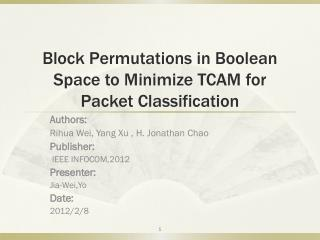DownloadDownload PresentationBlock Permutations in Boolean Space to Minimize TCAM for Packet Classification

# Block Permutations in Boolean Space to Minimize TCAM for Packet Classification

Télécharger la présentation## Block Permutations in Boolean Space to Minimize TCAM for Packet Classification

- - - - - - - - - - - - - - - - - - - - - - - - - - - E N D - - - - - - - - - - - - - - - - - - - - - - - - - - -
##### Presentation Transcript

1. Block Permutations in Boolean Space to Minimize TCAM for Packet Classification Authors: Rihua Wei, Yang Xu ,H. Jonathan Chao Publisher: IEEE INFOCOM,2012 Presenter: Jia-Wei,Yo Date: 2012/2/8

2. Introduction Ternary Content Addressable Memories (TCAMs) have been widely used to implement packet classification because of its parallel search capability and constant processing speed.

3. Introduction • Propose a novel technique called Block Permutation (BP) to compress the packet classification rules stored in TCAMs Rule r1, both the source port and destination port contain a range [1,5]. So both of them needs to be expanded to three prefixes, i.e., “001”, “01*”, “10*”. The combination of the prefix specifications of the two ranges will consume 3x3=9 TCAM entries, causing the well-known range expansion problem.

4. Relative work

5. Relative work In Figure 3 (b) spread sparsely and no two neighboring rule elements have the same action; thus, there are no two elements in the Karnaugh table that can be directly merged using logic optimization.

6. Block Permutation 01- - <> 11- - Ex : 0110 Ex’: 1110 B1 : 0001 B1 : 0001 B2 : 1101 B2’: 0101 B3 : 0010 => B3 : 0010 => B1 and B2’ merge to B6 B4 : 1110 B4’: 0110 B3 and B4’ merge to B7 B5 : **** B5 : ****

7. Block Permutation

8. Terms and Concepts 1. Block size :The size of a block is defined as the number of points that are contained in the block. For example, the size of the block “0**1” is 4. 2. Distance :The number of different counterpart bits in their Boolean representations. For example, the distance between the two points “0001” and “1101” is 2. EX: “0*01” and “01*0” is 1 , “0*01” and “0101” is 0. 3. Direction :If the Boolean representations of two blockshave wildcards(don’t care bit) that all appear in the same bit positions, we say these two blocks are in the same direction. EX: “0*01” and “0*10” in the same direction.

9. Terms and Concepts B6 and B 7 are target block. Target Blocks and Assistant Blocks: A pair of target blocks is the two blocks that we target to merge by a permutation.

10. Terms and Concepts Exchange row 10 and 11 To merge this target, we perform the operation “--10<>--11” over other two blocks “**10” and “**11”. These two blocks is the corresponding assistant.

11. Classifier compression Wp : assistant block size tar : target block p : permutation

12. Classifier compression • - -0 <> - - -1 (assistant block size : 3) • Target block : (distance : 2) • B6 : 0*01 => B6’ : 0*00 • B7 : 0*10 => B7’ : 0*11 • Can’t merge. 1. GET_TARGET : Try to find out all possible targets.

13. Classifier compression 2. EVAL_PERM :Have two tasks. One is to search all possible permutations for the targets we have obtained in previous step. The other is to determine if these permutations are worth performing and which permutation can yield the largest compression with the least overhead. Select the “best” one to perform : the number of blocks reduced minus the number of new blocks caused by the splitting of existing blocks.

14. Classifier compression • - 00 <> - - 01 • B4 : 1111 1111 • 1101 => 1100 produce two new small block and B4 disappears • B3 : 1100 1101 • => Invalid

15. Classifier compression 3. PERFORM : perform the permutation that has been selected in the step of EVAL_PERM to merge the target blocks.

16. Transformation implementation Use the pipeline structureto implement a series of transformations. If there are N transformations, we will design an N-stage pipeline. The one - block structure (one – stage pipeline)normally requires much less hardware resource than the pipeline structure, normally the stage has to be very complicated, thus largely reduce working speed. Propose a solution called stage-groupingto reduce the number of stages to trade-off between the speed and the cost.

17. Transformation implementation

18. Experiment Linux workstation driven by Intel Xeon 2.0GHz E5335 CPUs. Implemented the corresponding transformations by using the FPGA of Altera Cyclone III. The FPGA synthesis tool used is Quartus II. The reason why we chose Altera Cyclone is due to its low price and appropriate clock rate. This kind of FPGA can run on a clock up to 400MHZ or even higher, which is enough for our targeted throughput of 100M packets per second. Nr = 150 , Wmax = 102 , Wmin = 54 , using C/C++ language.

19. Experiment